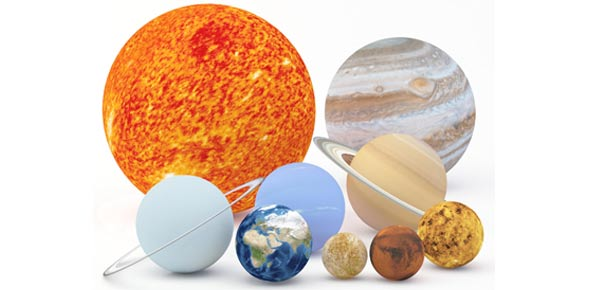# Astronomy Chapter 4-7 Quiz

52 Questions | Total Attempts: 78SettingsAstronomy is described as the study of science that deals with celestial objects, space and the whole universe. As we have finished up on studying chapter seven it is important to review what you understood so far from chapter 4 to 7. Try this quiz and jog your memory. All the best!

• 1.
About how many stars make up the Milky Way Galaxy
• A.

One, the Sun

• B.

Between 2 and 1,000,0000

• C.

Between 1,000,000 and 100,000,000

• D.

Between 100,000,000 and a billion

• E.

A few hundred billion

• 2.
Our understanding of the solar system has come in a way that can best be described as
• A.

• B.

Erractic

• C.

Steady until the last few decades, when, with the decline if the space program, we have learned little

• D.

We have learned more in the past few decades than during all the previous centuries combined

• 3.
Approxiamately what percentage of the entire solar system is contained in the sun
• A.

Less than 20%

• B.

35%

• C.

50%

• D.

75%

• E.

Over 99%

• 4.
One astronomical unit is
• A.

The distance across the solar system

• B.

The time requires for light to travel all the way around the Earth's orbit

• C.

The average distance from Earth to Sun

• D.

93 million seconds

• E.

The distance Earth travels around the Sun in one year

• 5.
Which of the following are the terrestrial planets
• A.

Mercury, Venus, Earth, Mars, Pluto

• B.

Earth, Mars, Jupiter, Saturn

• C.

Earth only

• D.

Mercury, Venus, Earth, Mars

• 6.
Which of the following ojects will never collide with Earth
• A.

Asteriods

• B.

Comets

• C.

Meteoroids

• D.

None of the above

• E.

All of the above

• 7.
Another term commonly used for meteor is
• A.

Dirty snowball

• B.

Evening star

• C.

Morning star

• D.

Falling star

• E.

Either B or C

• 8.
What causes a meteor shower
• A.

Earth crosses the orbit of a comet, and this orbit id littered with debris

• B.

Earth intercepts a stray swarm of asteriods

• C.

Earth encounters the asteriod belt

• D.

Earth collides with a meteor

• 9.
The orbits of most asteriods
• A.

Lie beyond the orbit of Mars

• B.

Cross the orbit of Mars

• C.

Cross the orbit of Earth

• D.

Lie beyond the orbit of Jupiter

• 10.
Comets are thought to reside mainly in
• A.

The inner solar system

• B.

Distant galaxies

• C.

The Oort Cloud and Kuiper Belt

• D.

Solar nebulae

• 11.
Which of the following has an icy compositon
• A.

Asteriods

• B.

The inner core of Saturn

• C.

Comets

• D.

Meteoriods

• E.

Moons of the terrestrrial planets

• 12.
Weak magnetic fields, slower rotation, and few moons characterize the__________planets
• 13.
The asteriod belt is located between the orbits of _______and ______
• 14.
The_________planets are relatively slow rotators, are dense and have few satellites
• 15.
A _______is body in the solar system which revolves around another larger object other than the Sun
• 16.
_______spend most of their time at the far edges of the solar system, only rarely passing into the iner solar system.
• 17.
The__________family of asteriods have orbits that take them closer to the Sun than the Earth
• 18.
Rocky debris, typically larger than 100 meters in diameter, seen orbiting the sun are_________
• 19.
Few meteors actually strike the Earth's surface, because most are ____and burn up in the atmosphere
• 20.
Small debris in the solar system less than 100 meters in diameter are called ____________
• 21.
The planets that, as a class, are the least densee are the __________planets
• 22.
One reason we infer that the earths core is metallic is that
• A.

The earth's average density is greater than the surface density

• B.

The earth's surface density is greater than the average density

• C.

Most of the continents are made of metals

• D.

Earthquakes can only occur if the core is metallic

• 23.
The age of the earth is estimated at roughly
• A.

4.6 thousands years

• B.

4.6 million years

• C.

4.6 billion years

• D.

Older than 10 billion years

• 24.
The earth's atmosphere helps to keep the surface warm by
• A.

Keeping out the cold space

• B.

Absorbing a large fraction of the incoming sunlight

• C.

Absorbing a large fraction of the incoming infrared

• D.

Reflecting sunlight back to space

• 25.
In estimatinf the earth's average age, we apply the technique of
• A.

Carbon-14 dating of organic materials

• B.Back to top